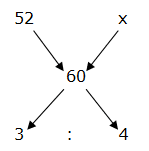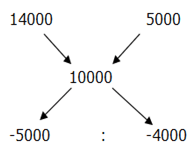# SBI Clerk Prelims 2020 Quantitative Aptitude Questions (Day-13)

Dear Aspirants, Our IBPS Guide team is providing new series of Quantitative Aptitude Questions for SBI Clerk Prelims 2020 so the aspirants can practice it on a daily basis. These questions are framed by our skilled experts after understanding your needs thoroughly. Aspirants can practice these new series questions daily to familiarize with the exact exam pattern and make your preparation effective.

[WpProQuiz 7651]

Ensure Your Ability Before the Exam – Take SBI Clerk 2020 Prelims Free Mock Test

Application Sums

1) In a group of 4 boys and 3 girls, 3 members are to be selected. In how many different ways can they be selected such that at least one boy should be there?

a) 56

b) 60

c) 34

d) 42

e) None of these

2) The first variety of rice priced at Rs. 52/kg is mixed with another variety of rice, which is in the ratio of 3 : 4. The mixed variety of rice is priced at Rs. 60/kg. Find the price of second variety of rice?

a) Rs. 58/Kg

b) Rs. 64/Kg

c) Rs. 62/Kg

d) Rs. 66/Kg

e) None of these

3) Pipe A and pipe B fill the tank in 30 hours and 20 hours respectively. If pipe A and B together opened simultaneously and after 10 hours Pipe B closed, in how many hour will pipe A take to fill the remaining tank?

a) 3 hours

b) 5 hours

c) 7 hours

d) 8 hours

e) None of these

4) The average salary of all the employees in a small company is Rs.10000. The average salary of 10 engineers is Rs.14000 and the average salary of the rest is Rs.5000. What is the total number of employees in the company?

a) 16

b) 17

c) 18

d) 19

e) None of these

5) A toy seller sold a toy offering a discount of 3% and earned a profit of 16.40%. What would have been the percentage of profit earned by the toy seller if no discount was offered?

a) 10%

b) 20%

c) 15%

d) 25%

e) None of these

6) A, B and C started a business by investing in the ratio of 6 : 7 : 10. After 5 months, A invested Rs. 10000 more but C withdraw Rs. 20000. Find the initial investment of B, if the share of A, B and C is in the ratio of 43 : 42 : 46?

a) Rs. 35000

b) Rs. 42000

c) Rs. 28000

d) Rs. 56000

e) None of these

7) The difference between the ages of Ranjit and Anvika, 2 years ago is 15 years. If the ratio of their ages, 7 years hence will be 3 : 2, then find the present age of Anvika’s mother, who is 25 years older than Anvika?

a) 50 years

b) 46 years

c) 44 years

d) 48 years

e) None of these

8) 30 men can complete the work in 12 days. After 5 days from the start of the work, some men left the job and the remaining work will be completed by remaining men in 10 days. Then find the total number of men left the job after 5 days of the work?

a) 10 men

b) 7 men

c) 9 men

d) 12 men

e) None of these

9) 150 m long train crosses another train of length 200 m in 42 sec, running in same direction. Find the speed of second train (In km/hr), if the speed of first train is 90 km/hr and the first train is faster than second one?

a) 70 km/hr

b) 60 km/hr

c) 50 km/hr

d) 80 km/hr

e) None of these

10) Out of the total monthly salary of Shyam, he spends 15 % on Rent, 9 % on travelling expenses and 20 % for his children’s education. Out of the remaining monthly salary, he spends 45 % for cosmetics and other expenses while the remaining salary is saved by him, which is equal to Rs. 21560. Find the monthly salary of Shyam?

a) Rs. 70000

b) Rs. 55000

c) Rs. 60000

d) Rs. 65000

e) None of these

Directions (1-10) :

Required number of ways

= > (4C1 and 3C2) or (4C2 and 3C1) or 4C3

= > (4 * 3) + (6 * 3) + 4

= >12 + 18 + 4 = 34According to the question,

(x – 60) / 8 = 3/4

x – 60 = 6

x = 66

The price of second variety of Rice = Rs. 66/Kg

LCM of (30, 20) = 60

Pipe A done the work in a hour = 2 units

Pipe B done the work in a hour = 3 units

A + B together fill the tank in 10 hours = 5 * 10 = 50 units

Remaining units of work = 60 – 50 = 10 units

A lone fill the remaining tank in = 10/2 = 5 hours

Let the total number of employees be x.

10000x = 14000 * 10 + 5000 * (x – 10)

=> 10000x = 140000 + 5000x – 50000

=> x = 90/5 = 18

(Or)= > 5 : 4

5’s = 10

1’s = 2

The total number of employees in the company = 9’s = 18

Let the C.P be Rs.100

Then, S.P = Rs.116.40

Let the marked price be Rs. x

Then, 97x/100 = 116.40

=> x = 120

Hence, S.P = Rs.120, C.P = Rs.100

So, Profit % = 20%

The share of A, B and C,

= > [6x * 5 + (6x + 10000) * 7] : [7x * 12] : [10x * 5 + (10x – 20000) * 7] = 43 : 42 : 46

= > [30x + 42x + 70000] : [84x] : [50x + 70x – 140000] = 43 : 42 : 46

= > [72x + 70000] : [84x] : [120x – 140000] = 43 : 42 : 46

According to the question,

(72x + 70000) / 84x = (43/42)

72x + 70000 = 86x

70000 = 14x

x = 70000 / 14 = 5000

The initial investment of B = 7x = Rs. 35000

2 years ago, Ranjit – Anvika = 15

7 years hence, The ratio of Ranjit and Anvika = 3 : 2 (3x, 2x)

Present age of Ranjit and Anvika = 3x – 7, 2x – 7

According to the question,

3x – 2x = 15

x = 15

Present age of Anvika = 2x – 7 = 23 years

Present age of Anvika’s mother = 23 + 25 = 48 years

Total work = 30 * 12 = 360 units

5 days work = 30 * 5 = 150 units

Remaining work = 360 – 150 = 210 units

Given,

210 / (30 – x) = 10

21 = 30 – x

x = 30 – 21 = 9

The total number of men left the job after 5 days of the work = 9 men

T = D / S

According to the question,

42 = (150 + 200) / [(90 – x) * (5/18)]

42 = (350 * 18) / [[(90 – x) * 5]

(350 * 18) / (42 * 5) = 90 – x

30 = 90 – x

x = 90 – 30 = 60

The speed of second train = 60 km/hr

Let the monthly salary of Shyam be x,

x * (56/100) * (55/100) = 21560

x = 21560 * (100 / 56) * (100 / 55) = 70000

The monthly salary of Shyam = Rs. 70000

 Check Here to View SBI Clerk Prelims 2020 Quantitative Aptitude Questions Day 12 Day 11 Day 10 Click Here for SBI Clerk 2020 – Detailed Exam Notification